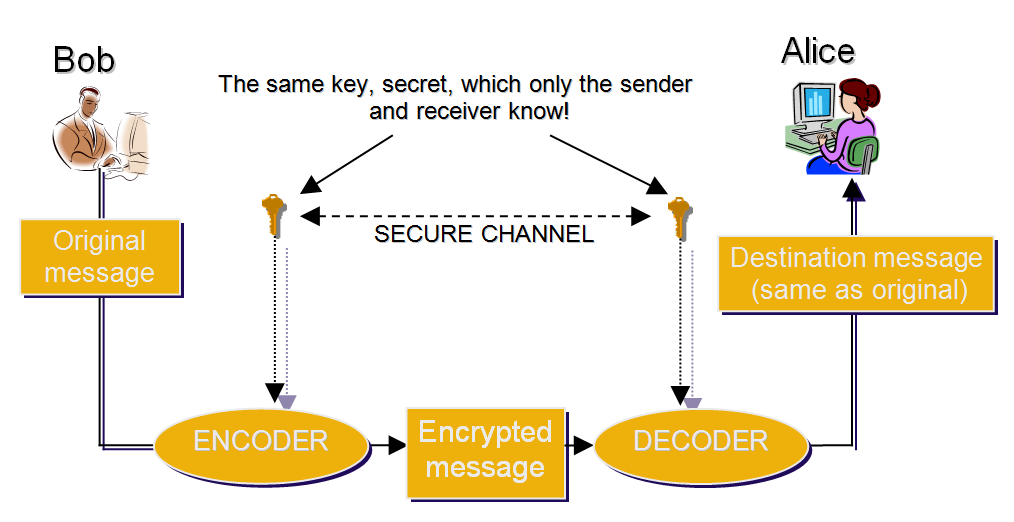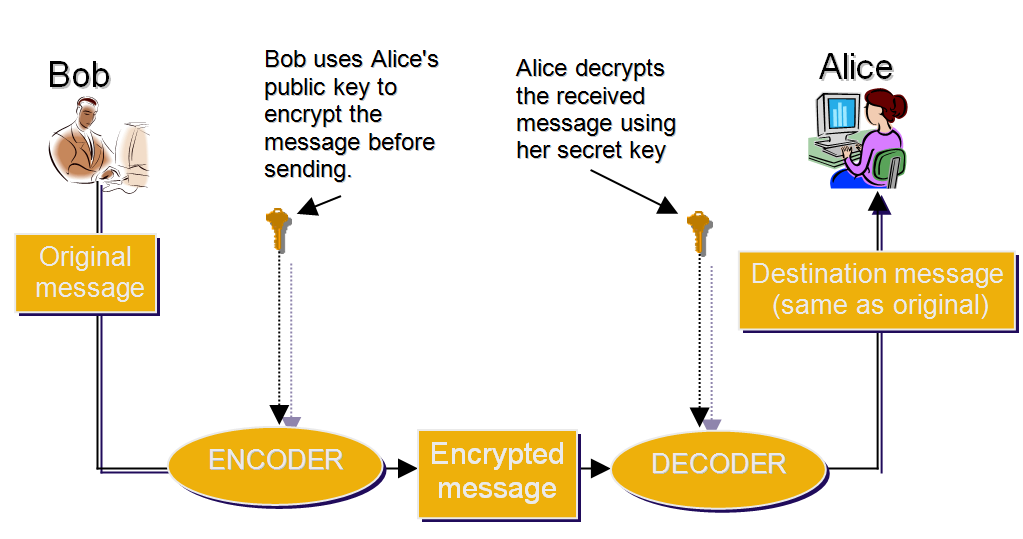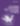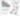Skip to main content

# A Deep Dive into Cryptography

Blockchain

Share:

#### Free JavaScript Book!Write powerful, clean and maintainable JavaScript.

RRP \$11.95

This Deep Dive into Cryptography was originally published at Bruno’s Bitfalls website, and is reproduced here with permission.

The media is jam-packed with content about cryptocurrency and everyone is raving about the importance of public and private keys. You’ve heard of encryption, but do you know what it actually is and how it works?

This post will take you back to the basics and explain encryption, describe the different types, and demonstrate algorithm examples, all in a newbie-friendly way. If you’ve ever wanted to understand this but it seemed too complicated, you’ll love this post.

## Cryptography

Cryptography is, in a nutshell, turning a message into such a format that it makes sense only to the recipient, and not anyone who might get their hands on it in between.

What is the problem we’re trying to solve?

Suppose there are two people who want to exchange encrypted messages, regardless of the communication channel they’re using — letters, SMS, email … They first have to come to an agreement about a set of rules to apply when encrypting or decrypting the message. These rules are a set of one or more functions which must have a counterfunction. That is, if a given function is used to encrypt a message, then there must exist a counterfunction to decrypt it. In mathematical parlance, such a function is bijective.

The sender of the message applies a function to a set of information and gets an encrypted version of this information which can then be sent to the recipient. The recipient applies the counterfunction to extract the true information out of the encrypted version of the message. If someone in the middle intercepts this communication but they don’t have the counterfunction with which to decrypt the message, they’ll be unable to read it.

### Alice and Bob

Let’s make this clearer with the help of a trivial example. Suppose Bob wants to send an SMS containing “I LOVE YOU” to Alice, but can’t risk it being seen by someone else. (Someone picking Alice’s phone up would see the message.) To successfully exchange encrypted messages, Alice and Bob need to come to an agreement about the way of encrypting/decrypting messages. Let’s assume this is the agreement:

Every letter in the message will be replaced with the double-digit index number of that latter in the English alphabet. “A” will be “01”, “B” will be “02”, etc. “00” will indicate a space character. This is their encrypt/decrypt function, and it’s bijective.

code: symbol code: symbol code: symbol
00: space 09: I 18: R
01: A 10: J 19: S
02: B 11: K 20: T
03: C 12: L 21: U
04: D 13: M 22: V
05: E 14: N 23: W
06: F 15: O 24: X
07: G 16: P 25: Y
08: H 17: Q 26: Z

Bob will therefore be sending the message:

``````09001215220500251521
``````

Should someone intercept this message, it won’t make much sense to them. Their love will remain a secret. Alice, on the other hand, will be able to easily decrypt it and blush.

## Symmetric Encryption (with a Private Key)

The above outlined approach is called symmetric private key encryption. “Symmetric” indicates that the message can be encrypted and decrypted using the same key (secret). The key (secret) is actually the letter-to-number-to-letter replacement function we described. It looks a little like this:A system like this might seem perfect at first glance. Of course, a more complex bijective function is required to make this communication truly secure. The system as such is perfectly safe for as long as Alice and Bob can meet and arrange a method of encryption/decryption beforehand. But what if, like in most cases today, the people communicating aren’t actually in close physical proximity, or maybe don’t even know each other? How can they safely exchange a secret key without risk of it falling into the wrong hands?

This is the biggest downside of such a shared key encryption: there has to be a pre-established secure channel via which to exchange the key.

### A Possible Solution

It’s clear that, unless a secure channel already exists, it’s nearly impossible to safely exchange the private key. If the channel did exist already, then there’s no need for another one.

The solution is not to find a safe way of exchanging the key, but to eliminate the need for such an exchange altogether. This can be accomplished by adding another key into the mix. One of them would be used only for encrypting, the other for decrypting.

The encrypting key could be available to everyone. In fact, it must be available to everyone, because without it it’s impossible to encrypt messages and send them to the recipient. This key is called the public key.

The other key is used only for decrypting and shouldn’t be sent to anyone. Only the recipient of encrypted information has it, and we call this one the private key.

## Asymmetric Encryption (with a Public Key)Looking at the image above, we can see that there’s no need for a secure channel to exchange keys through. The asymmetry is in the fact that it’s impossible to encrypt and decrypt the message with the same key. A separate one is needed for each action.

If Bob wants to send Alice an encrypted message, he has to have her public key. This public key could be given to him directly by Alice, or she could just publish it on her website where anyone who wants to send her an encrypted message can find it. When Alice receives the message encrypted with her public key, she uses the private key to decrypt it, which makes the message readable again.

If Alice wants to send a reply, she now needs Bob’s public key. The procedure is identical: she encrypts the message, sends it, and only Bob can decrypt it with his private key.

It’s important to note that to make this kind of communication feasible, the keys need to be generated with procedures complex enough to offset any computer’s ability to guess them for an unreasonable amount of time.

### How does it work?

If you don’t fully understand the terms in the section below, don’t worry. Read through them superficially; they’ll be explained on an example immediately afterwards.

To make it impossible to decrypt a message with only the public key, or to derive the private key from the public key, one-directional mathematical functions are used. A one-directional function is such that `f(x)` can be calculated for any `x` but not the other way around. For example, if we know the sum is 950, we can’t guess which numbers we’ve summed to get it because the number of possible combinations is infinite.

There are many algorithms to calculate such functions. We’ll demonstrate one such algorithm below: it’s called the RSA algorithm, based on the initials of the names of its creators in 1977 (Ron Rivest, Adi Shamir, Leonard Adleman).

1. Select 2 primary numbers — numbers divisible only by 1 or themselves. For example:

• p = 61
• q = 53
2. Multiply them `n = p x q`:
`n = 61 x 53 = 3233`

3. Calculate the lowest common multiple `λ(n) (p-1) i (q-1)`:
`nzv (p-1,q-1) = nzv (60,52) = 780`. This can be done with the algorithm presented here.

4. Pick any number between 1 and the multiple calculated previously, so that this number is relatively prime or coprime to the initial two numbers. Numbers are coprimes if the only positive number that divides both of them is 1. In this case, that’s `e = 17`.

5. Calculate the modular multiplicative inverse of `e(mod nzv)`. We look for `d` such that:

• `(d x e) mod nzv = 1`
• `(d x 17) mod 780 = 1`
• `d = 413`
6. These calculations produce all the necessary components of a public and private key set. The public key is the pair `(n, e)` and the private key is `(n, d)`. Thus, the public key is `(n = 3233, e = 17)`. The public key serves to encrypt the messages via the following formula:

c(m)=m17 mod 3233

The private key is `(n=3233, d=413)`. It’s used to decrypt a message:

m(c)=c413 mod 3233

Now that we have the encryption and decryption functions, we can come back to our Alice and Bob scenario. Since these functions can only process numbers, we need to turn letters into numbers first. The ASCII table — a standard used by computers when working with letters on computer systems — can come in handy. Let’s only focus on uppercase letters here.

code: symbol code: symbol code: symbol
32: space 73: I 82: R
65: A 74: J 83: S
66: B 75: K 84: T
67: C 76: L 85: U
68: D 77: M 86: V
69: E 78: N 87: W
70: F 79: O 88: X
71: G 80: P 89: Y
72: H 81: Q 90: Z

For every ASCII code from Bob’s message, we need to calculate the `c(m)`. As the message is “I LOVE YOU”, the ASCII of the first letter is 73. `c(73)` is:

c(m) = m17 mod 3233
c(73) = 7317 mod 3233
c(73) = 47477585226700098686074966922953 mod 3233
c(73) = 1486

Let’s also calculate the rest.

Space: c(32) = 3217 mod 3233 = 1992
L: c(76) = 7617 mod 3233 = 2726
O: c(79) =7917 mod 3233 = 1307
V: c(86) = 8617 mod 3233 = 1906
E: c(69) = 6917 mod 3233= 28
Y: c(89) = 8917 mod 3233 = 99
U: c(85) = 8517 mod 3233 = 2310

The encrypting function calculates the `mod 3233` (division remainder when dividing with 3233) so the result of a letter’s encryption can’t be more than 3232, which in turn means four is the maximum number of digits in each letter’s encrypted version. Therefore, we pad each number with zeroes on the left side: 1486, 1992, 2726, 1307, 1906, 0028, 1992, 0099, 1307, 2310.

The full sendable message is:

``````1486199227261307190600281992009913072310
``````

Alice has her private key, and can use it to decrypt this. She’ll use the previously defined inverted function m(c)=c413 mod 3233 where `c` is the encrypted message. The inverted function shows that `mod 3233` is being calculated, and that every letter can be at most 3232 and have four digits. Thus, Alice knows the message needs to be divided into sets of four, the leading zeros of each being meaningless:

``````1486 1992 2726 1307 1906 (00)28 1992 (00)99 1307 2310
``````

Let’s decrypt 1486:

m(c)=c413 mod 3233
m(1486) = 1486413 mod 3233
m(1486) = 1,1060335282256977039647849058382e+1310 mod 3233
m(1486) = 73

We got the number 73, which according to the ASCII table matches the letter “I”. By following the same process, we can decrypt the rest of the message:

m(1992) = 1992413 mod 3233 = 32, ASCII 32 = razmak
m(2726) = 2726413 mod 3233 = 76, ASCII 76 = L
m(1307) = 1307413 mod 3233 = 79, ASCII 79 = O
m(1906) = 1906413 mod 3233 = 86, ASCII 86 = V
m(28) = 28413 mod 3233 = 69, ASCII 69 = E
m(1992) = 1992413 mod 3233 = 32, ASCII 32 = razmak
m(99) = 99413 mod 3233 = 89, ASCII 89 = Y
m(1307) = 1307413 mod 3233 = 79, ASCII 79 = O
m(2310) = 2310413 mod 3233 = 85, ASCII 85 = U

Let’s re-iterate why asymmetric encryption is better than symmetric encryption. With symmetric encryption, there’s only one key which is used to both decrypt and encrypt messages. If participants want to communicate securely, they first need to exchange that key safely. If they’re physically remote, this becomes a big problem. With asymmetric encryption, there’s a public key which we can freely give out, and which people can use to encrypt messages intended for us. Our private key remains secret, and is used only to decrypt the messages we receive. There’s no need for a secure channel via which to exchange a secret key, making asymmetric encryption much safer than symmetric encryption.

But would it be possible to calculate the private key from the public key?### Guessing the Private RSA Key

Let’s look at the functions one more time:

• Encrypt: F(x)=xe mod (p x q)
• Decrypt: F-1(c)=cd mod (p x q)

The encryption function — that is, the `(p x q, e)` pair — represents a public key. If we know this pair and we want to calculate the private key, we need to find the numbers `p` and `q`. That means we need to factorize the product of `p` x `q`. If we assume the numbers `p` and `q` are 1024 bit numbers, then their product is a 2048 bit number — a number with 617 digits if represented as a decimal number. To factorize such a big number, even today’s most powerful supercomputer would need an absurd amount of time, making the process a mathematical impossibility.

It’s not technically impossible to factorize the number. There are special algorithms developed for that exact purpose, and the most efficient one right now is GNFS (General Number Field Sieve). It’s especially handy for factorization of numbers with more than 110 digits. The table below lists the time required to factorize a big number expressed in MIPS (Million Instructions per Second). Accordingly, one MIPS-year is the number of computer operations executable in one year by a computer with the power of 1 MIPS. That number is 3.1536 x 1013.

Key length MIPS-years needed to factorize it
512-bitni 30.000
768-bitni 200.000.000
1024-bitni 300.000.000.000
2048-bitni 300.000.000.000.000.000.000

If we assume that the applied algorithm multiplies 1024 bit numbers, producing a 2048 bit product and that today’s most powerful personal computer CPUs have around 300,000 MIPS, the number gets pretty high. Even today’s most powerful quantum computers with 100 million MIPS of power (which are a rarity, if they even exist), would need 3,000,000,000,000 years to factorize a 2048 bit number.

### Mersenne primes

Given that big prime numbers are the main idea behind the RSA algorithm, it’s increasingly more important to find big primes. There’s a subclass of primes called Mersenne primes, and they look like this: 2n-1. They were named after a French friar who was the first to (wrongly) identify 11 of them. There’s a big movement around finding the biggest possible Mersenne primes these days. (You can find the details at Mersenne.org.) The last (49th) and biggest Mersenne number so far was found on January 7th, 2016. It’s 274.207.281-1 and has 22,338,618 digits. The one before it was found on January 1st 2013: it was 257.885.161-1 and has almost 5 million fewer digits than the 49th number.

One more fun fact about prime numbers: the EFF, Electronic Frontier Foundation, has bounties on big primes. Their website lists these bounties, and shows that the \$50,000 reward for finding a 1-million-digit prime and the \$100,000 reward for finding a 10-million-digit prime were given away, with the \$150,000 and \$250,000 rewards for 100 million and 1 billion digits respectively are still pending. Got a good algorithm idea? Maybe you can be the one to find the number!

## Asymmetric Encryption and Bitcoin

So what does all this have to do with cryptocurrency, other than sharing the “crypto” name?

While the below applies to almost all cryptocurrencies, let’s take the most famous one — Bitcoin — as an example. Bitcoin also uses a public/private key pair. The public key — or at least a form of it — is the address to which you can send some Bitcoins. Like with encrypted messages where a public key is needed so that someone can send us a message, with Bitcoin a public key is needed so that someone can send us money. On the other hand, a private key lets us confirm, approve, and perform a transaction with which we send some of our bitcoins from our address (account) to someone else’s public key (address).

The idea is similar, but Bitcoin has a different approach at calculating the private and public key. The private key of Bitcoin is a 256-bit number. We’re generally dealing with a big number randomly picked from a set of 2256 numbers. That’s a little over 1077 possible choices. This might not seem like much, but considering the estimation that there are 1080 atoms in the whole universe, the size of this number can still make us pause and think. Just counting all those numbers at a speed of one billion per second would take more time than the age of the universe.

So we have a private key which only we know and which can’t be guessed by anyone or any computer in a reasonable amount of time. In the Bitcoin protocol, the private key is used to calculate the public key using the ECC algorithm, Elliptic-curve cryptography. This algorithm is based on a curve the function of which can be mathematically expressed as y2=x3+ax+b. The result is the public key. This URL and this URL explain the algorithm in detail if you’re interested.

With Bitcoin, the public key isn’t the address to send money to, but it can be easily calculated using the following formula:

``````address = RIPEMD160 (SHA256 (public key))
``````

This URL contains details about the process if you’re interested.

## Conclusion

It’s important to remember the difference between encoding and encrypting. Encoding is the process of making the sent message as identical to the original as possible to minimize errors. Encrypting is making the message unreadable to everyone but the intended recipient.

There are several different kinds of encryption, the main ones being asymmetric and symmetric. We covered the former in this article, and explained that in contrast with symmetric encryption, asymmetric encryption introduces a public and private key rather than just one private key shared between the parties, making it possible to securely communicate across insecure channels.

The advantage of asymmetric encryption becomes obvious in cryptocurrency, where the public key is used to receive funds and check balance and transactions, whereas the private key is the only way to actually sign messages and send the tokens.### Bruno Skvorc

Bruno is a blockchain developer and technical educator at the Web3 Foundation, the foundation that's building the next generation of the free people's internet. He runs two newsletters you should subscribe to if you're interested in Web3.0: Dot Leap covers ecosystem and tech development of Web3, and NFT Review covers the evolution of the non-fungible token (digital collectibles) ecosystem inside this emerging new web. His current passion project is RMRK.app, the most advanced NFT system in the world, which allows NFTs to own other NFTs, NFTs to react to emotion, NFTs to be governed democratically, and NFTs to be multiple things at once.

#### New books out now!Learn the basics fo programming with the web's most popular language - JavaScriptA practical guide to leading radical innovation and growth.

### Latest Remote Jobs